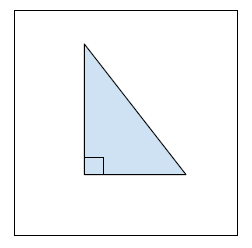# Find the hypotenuse of a right angled triangle with given two sides in C++

In this problem, we are given two integer values H and B defining height and base of a right angled triangle. Our task is to find the hypotenuse of a right angled triangle with given two sides.

Right Angled Triangle is a special triangle whose two angles are at right angles.Let's take an example to understand the problem,

Input : B = 5, H = 12
Output : 13.00

## Solution Approach

A simple solution to the problem is using the concept of pythagoras theorem to find the hypotenuse of a triangle using the base and height.

Pythagoras Theorem States that the square of the hypotenuse of the right angled triangle is equal to the sum of squares of the other two sites of the triangle.

Formulated as −

$H^2\:=\:h^2\:+\:b^2$

## Example

Program to illustrate the working of our solution

#include <iostream>
#include <math.h>
using namespace std;
double findHypotenuseTriangle(double h, double b) {
return ( sqrt((h*h) + (b*b)) );
}
int main() {
double h = 5.0, b = 12.0;
cout<<"Base of right angled triangle "<<b<<endl;
cout<<"Height of right angled triangle "<<b<<endl;
cout<<"Hypotenuse of right angled triangle = "<<findHypotenuseTriangle(h,b);
return 0;
}

## Output

Base of right angled triangle 12
Height of right angled triangle 12
Hypotenuse of right angled triangle = 13